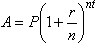# 5 Benefits Of Using A Mutual Funds Lumpsum Calculator

Last Updated on

There are basically two types of investments in mutual funds schemes. Systematic Investment Plans (SIP) in mutual funds and mutual funds lumpsum investment. In SIP, one has to make a nominal investment over recurring intervals whereas in lumpsum investment the investment is made in one go with a lumpsum amount.

A mutual funds lumpsum calculator will help you plan your investments with a better analysis. A mutual funds lumpsum calculator calculates the maturity amount of a given mutual fund investment as it would be after a given period of time. With the help of the mutual fund lumpsum calculator, one can have prior knowledge about the amount they will be having after the maturity period. One only needs to enter the investment amount, the expected rate of returns and the period for which the investment has been made and they can get the desired output from the lumpsum calculator online.

## Lump-Sum Calculator Formula

Though one may come across different mutual fund lumpsum calculator online, all of them follow a common formula to compute the maturity value of the principal amount in any mutual fund investment.

The said formula is Lump Sum Mutual Fund Calculator Formula =>A = P (1 + r/n) ^nt

Here the characters represent:

A = Estimated Return (Final Value)

P = Present Value

r = Rate of Return

t = Duration of Investment (months or years)

n = Number of Compounded Interest In years.

As mutual funds are subjected to market risk, the amount that comes after applying the aforementioned formula would be slightly different from the actual amount.

The lumpsum calculator, however, will give an approximate analysis of the investment performance over a given period.

## Benefits Of Mutual Funds lumpsum Calculator

A mutual funds lumpsum calculator provides many advantages that will give your investment a better direction and effective analysis. Some of these advantages are given below:

1. You can calculate the return value of your investment and the amount you will be gaining every year. This will make you see how your funds are faring in the market. If they are maintaining stability over a couple of years, you can plan your future accordingly with an expected maturity amount to be had once the investment period is over.

2. What with compound interests and market fluctuations, it may become quite difficult to compute the maturity amount. Also, the complex mathematical formula may pose a difficulty to many regarding the calculation. A mutual funds lumpsum calculator, on the other hand, will provide a hassle-free and simple output without needing to wrack one’s hand with the formula.

All you have to do is to insert the requisite values which are the lumpsum investment amount, the interest rate applied on the said amount and the period for which the amount has been invested. Based on the yearly performance, you can ascertain what the revised maturity amount would be like after each successive year.

3. Accuracy is another factor that makes the lumpsum calculator a great option for calculating mutual fund investment returns. The calculator is solely designed for this purpose and the output it will render will be effectively giving you data on which you can rely. Since mutual funds are subject to market risks, it is not altogether correct to expect that the lumpsum calculator will give an exact amount that you are going to have after the end of the investment period.

What the calculator rather does is based on the current interest rate, it provides an estimate of the final amount to be had provided the rates do not fluctuate. Even so, the final amount the calculator generates will not be far off from the actual amount. So, in other words, you can totally rely on it.

4. As the calculator is a perfect tool for calculating the mutual funds lumpsum investment returns, you will have in the distant future, it helps in making other investments. What this implies is that based on the performance of your current lumpsum mutual funds investment and the returns it will generate, you can plan your future or retirement period accordingly. Along with that, it will also establish for you whether it is good or not to go for further investment and what they should be like.

5. The interface of the lumpsum calculator is very user friendly. Being simple and yet an effective tool, it encourages the investors to seek it for their investment analysis. As only necessary options and details are given on the online mutual funds lumpsum calculator, the investor or a potential investor can easily figure out how to operate it. The ease of accessibility is an encouraging factor for many to make a wise decision regarding their future investment strategies.Thus, the mutual fund lumpsum calculator can come off handy to many investors who have already invested in some mutual funds scheme to keep track of their investment performance. Also, the calculator plays an important role for the potential investors who may want to figure out what returns they can expect on a certain amount of lumpsum investments.